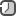### Introduction to Android screen densities

Screen density is a ratio of resolution and display size, which can be quantified as dots per inch, or dpi. The higher the dpi, the smaller each individual pixel is, and the greater clarity.

Pixels is the most granular unit of measure. Pixel density refers to how many pixels have been squeezed into a physical amount of space (often an inch). Something like 1920x1080, 2560x1440, etc.

DPI is dots per inch unit and helps to measure the size of a pixel. It is an indicative of how many pixels can be found in one inch and is calculated by dividing the number of pixels across or down a screen by the width or height in inches. A higher DPI means each individual pixel must be smaller and smaller in order to be able to fit into the available space, meaning the clearer the screen will be and the higher the level of detail that screen can draw. Simply put, a higher DPI means more detail is displayed per inch, but does not necessarily correlate with a higher screen resolution.DP is the density-independent pixel unit. One DP is one pixel on a 160 DPI screen. Independent pixel because the unit of measure is independent of the density, it is independent of the screen dimension and resolution. Whether the density is high or low, the elements on the screen will have approximatively the same size/look. The density-independent pixel is equivalent to one physical pixel on a 160 DPI screen, the baseline density assumed by the platform (as described later in this document). At run time, the platform transparently handles any scaling of the DP units needed, based on the actual density of the screen in use.

The formulas for calculation are following

px = dp * (dpi/160)

dp = (px * 160) / dpi

or use converter.

For example, on 240 DPI screen, 1 DP would equal 1.5 physical pixels. Using DP units to define your application’s UI is highly recommended, as a way of ensuring proper display of your UI on different screens.

In Android, user interfaces can be created in xml, and programmatically in code. In order to express a form of distance or length, there are several units for dimensions. These can be used on elements to set widths, heights, margins, padding, and more.

• px - an actual pixel on the screen. This is a density dependent unit, and the physical size of a single px varies depending on screen density.
• in - a physical inch on the screen. This is a density independent unit, and the physical size of a single in is the same on every screen density. The number of pixels a single in translates to varies depending on screen density.
• mm - a physical millimeter on the screen. This is a density independent unit, and the physical size of a single mm is the same on every screen density. There are 25.4 mm in an inch. The number of pixels a single mm translates to varies depending on screen density.
• pt - a point, a common font size unit, on the screen. This is a density independent unit, and the physical size of a single pt is the same on every screen density. There are 72 pt in an inch. The number of pixels a single pt translates to varies depending on screen density.
• dp - a density independent pixel. This is a density independent unit, however the physical size of a single dp is only approximately the same on every screen density. There are approximately 160 dp in an inch. A scaling factor, depending on the density bucket of the device, is applied to convert dp to the number of pixels at 160 dpi. The number of pixels a single dp translates to varies depending on the pixel on screen density and the density bucket the device falls into.
• sp - a scale independent pixel, specially designated for text sizes. One SP is one pixel on a 160 DPI screen if the user's global text scale is set to 100% This is a density independent unit, however the physical size of a single sp is only approximately the same on every screen density. Scaling factors, depending on the density bucket of the device, as well as the user’s text size preference, are applied to convert sp to the number of pixels at 160 dpi. The number of pixels this translates to varies depending on screen density and the density bucket the device falls into. .Let's look at code for getting Screen Density and Screen DensityDPI, width and height of the android device using `DisplayMetrics`.

`DisplayMetrics` is a structure describing general information about a display, such as its size, density, and font scaling.

```public class DeviceDimensionsHelper {
// DeviceDimensionsHelper.getDisplayWidth(context) => (display width in pixels)
public static int getDisplayWidth(Context context) {
DisplayMetrics displayMetrics = context.getResources().getDisplayMetrics();
return displayMetrics.widthPixels;
}

// DeviceDimensionsHelper.getDisplayHeight(context) => (display height in pixels)
public static int getDisplayHeight(Context context) {
DisplayMetrics displayMetrics = context.getResources().getDisplayMetrics();
return displayMetrics.heightPixels;
}

// DeviceDimensionsHelper.convertDpToPixel(25f, context) => (25dp converted to pixels)
public static float convertDpToPixel(float dp, Context context){
Resources r = context.getResources();
return TypedValue.applyDimension(TypedValue.COMPLEX_UNIT_DIP, dp, r.getDisplayMetrics());
}

// DeviceDimensionsHelper.convertPixelsToDp(25f, context) => (25px converted to dp)
public static float convertPixelsToDp(float px, Context context){
Resources r = context.getResources();
DisplayMetrics metrics = r.getDisplayMetrics();
float dp = px / (metrics.densityDpi / 160f);
return dp;
}
```